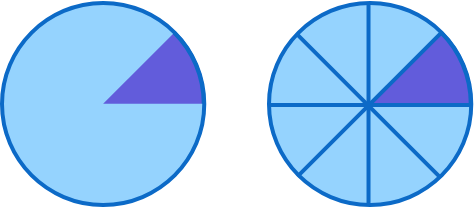Back

## A Different Sort of Pie Chart

Fractions are often represented as pie charts, where a circle is broken into slices called sectors.We can use the relationship between fractions and circle sectors to calculate the area of the sectors. For example, the central angle of the dark-shaded sector above is one-eighth of the circle, so its area is $\frac18$ the area of the circle.

But what happens if—instead of cutting a circle up—we stretch it out and multiply its area?